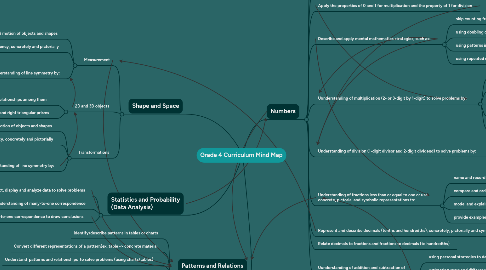# Grade 4 Curriculum Mind Map

Get Started. It's FreeGrade 4 Curriculum Mind Map## 2. Shape and Space

### 2.1. Measurement

2.1.1. Describe and analyze position and motion of objects and shapes

2.1.2. Demonstrate an understanding of congruency, concretely and pictorially

2.1.3. Demonstrate an understanding of line symmetry by:

2.1.3.1. Identifying symmetrical 2D shapes

2.1.3.2. Creating symmetrical 2D shapes

2.1.3.3. drawing one or more lines of symmetry in a 2D shape

### 2.2. 2D and 3D objects

2.2.1. Describe characteristics of 3D objects and 2D shapes and analyze relationships among them

2.2.2. Describe and construct right rectangular and right triangular prisms

### 2.3. Transformations

2.3.1. Describe and analyze position and motion of objects and shapes

2.3.2. Demonstrate an understanding of congruency, concretely and pictorially

2.3.3. Demonstrate an understanding of line symmetry by:

2.3.3.1. Identifying symmetrical 2D shapes

2.3.3.2. creating symmetrical 2D shapes

2.3.3.3. Drawing one or more lines of symmetry in a 2D shape

## 4. Numbers

### 4.3. Understanding of addition of numbers to 10 000 and their corresponding subtractions (3 and 4 digit numerals) by:

4.3.1. using personal strategies for adding and subtracting

4.3.2. estimating sums and differences

4.3.3. solving problems involving addition and subtraction

### 4.5. Describe and apply mental mathematics strategies, such as:

4.5.1. skip counting from a known fact

4.5.2. using doubling or halving & adding or subtracting one more group

4.5.3. using patterns in the 9s facts

4.5.4. using repeated doubling to determine basic multiplying facts to 9 × 9 and related division facts

### 4.6. Uunderstanding of multiplication (2- or 3-digit by 1-digit) to solve problems by:

4.6.1. using personal strategies for multiplication with/without concrete materials

4.6.2. using arrays to represent multiplication

4.6.3. connecting concrete representations to symbolic representations

4.6.4. estimating products

4.6.5. applying the distributive property

### 4.7. Understanding of division (1-digit divisor and 2-digit dividend) to solve problems by:

4.7.1. using personal strategies for dividing with and without concrete materials

4.7.2. estimating quotients

4.7.3. relating division to multiplication

### 4.8. Understanding of fractions less than or equal to one or use concrete, pictorial and symbolic representations to:

4.8.1. name and record fractions for the parts of a whole or a set

4.8.2. compare and order fractions

4.8.3. model and explain that for different wholes, two identical fractions may not represent the same quantity

4.8.4. provide examples of where fractions are used

### 4.11. Uunderstanding of addition and subtraction of decimals (limited to hundredths) by:

4.11.1. using personal strategies to determine sums and differences

4.11.2. estimating sums and differences

4.11.3. using mental mathematics strategies to solve problems